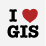1170
7
07-17-2018 07:25 AMOccasional Contributor

You provide a nice example of how to set up calculatestatistics here:

However, most folks (me) are interested in the actual values produced.

Can someone lead me in a direction to get an actual value from IReadOnlyList<TableStatisticsResult>?

I calculated a SUM of a field, but want to provide the value to a new variable for further calculation...

Thank You.

1 Solution

Accepted SolutionsbyEsri Regular Contributor

Hi Brian,

Try this:

``var value = vlsStatsResult.First().StatisticsResults.First().Max;‍``

Thanks

Uma

7 RepliesOccasional Contributor III

Yes, I have exactly the same question.  I think I've been able to calculate the MAX value of a certain field, but I have no idea of how to actually get the value out of the TableStatisticsResult.

Any help is appreciated.

``````FeatureClass vlsFC = vlsLayer.GetFeatureClass();
FeatureClassDefinition fcd = vlsFC.GetDefinition();

Field fldVLS_NO_1 = fcd.GetFields().First(x => x.Name.Equals("VLS_NO_1"));
StatisticsDescription vlsMaxDesc = new StatisticsDescription(fldVLS_NO_1, new List<StatisticsFunction>() { StatisticsFunction.Max });
TableStatisticsDescription tsd = new TableStatisticsDescription(new List<StatisticsDescription>() { vlsMaxDesc });

//This does not work
ArcGIS.Desktop.Framework.Dialogs.MessageBox.Show("Max VLS is " + vlsStatsResult.Max);‍‍‍‍‍‍‍‍‍‍``````byEsri Regular Contributor

Hi Brian,

Try this:

``var value = vlsStatsResult.First().StatisticsResults.First().Max;‍``

Thanks

UmaOccasional Contributor III

Great!  Thanks Uma.byEsri Regular Contributor

To answer Mike's original question, I've expanded the snippet he posted to extract the calculation results. Here is the code:

// Calculate Statistics

foreach(TableStatisticsResult tableStatisticsResult in tableStatisticsResults)
{
// Get the Region name
// If multiple fields had been passed into TableStatisticsDescription.GroupBy, there

// would be multiple values in TableStatisticsResult.GroupBy
string regionName = tableStatisticsResult.GroupBy.First().Value.ToString();

// Get the statistics results for the Population_1990 field
StatisticsResult pop1990Statistics = tableStatisticsResult.StatisticsResults;
double population1990Sum = pop1990Statistics.Sum;
double population1990Average = pop1990Statistics.Average;

// Get the statistics results for the Population_2000 field
StatisticsResult pop2000Statistics = tableStatisticsResult.StatisticsResults;
double population2000Sum = pop2000Statistics.Sum;
double population2000Average = pop2000Statistics.Average;

// Do something with the results here...

}

I hope this helps.Occasional Contributor

Thanks, Uma.

".StatisticsResults.First().Max;" was all I needed to get moving again on my code.

Thanks, Rich for the expanded clarification.Occasional Contributor

Coda to the original question:

How can I perform Table operations like .CalculateStatistics upon a FeatureLayer.GetSelection()?

i.e., converting a featurelayer selection (from user) into a temporary table so I can .CalculateStatistics.byEsri Regular Contributor

Hi Mike,

There is no direct way to do this.  The best solution at this point is to create a temporary table from your selection using geoprocessing tools and use that to run Table.CalculateStatistics().